# Two fair dice are tossed, and the face on cach de is oberved. Use a tree disgram to find the 36 ample points coetained in the sample spoce.b Assign probabities te the sample points in purtae Find the peobubity of cach of the following eventsA-(3 showing on cach die)B- (m of two mumbers shouing is 7)C- (um of rwo numbers showing is evem)d. Also, for evets A, B, andC. which one is simple event, which one is cempoundevent?e Event D- A. Event D and event C independent or not? Event D and event Bmutually exclusive or notf. Consider event D and event C, Find the probability of cach of the followingevents p(Dn) P(DUC), p(DIC).p(C'iD').p(D'nc)

Question
6 views

A&B&C&D&E&F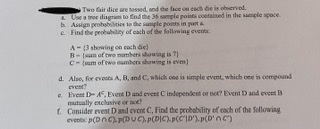help_outlineImage TranscriptioncloseTwo fair dice are tossed, and the face on cach de is oberved . Use a tree disgram to find the 36 ample points coetained in the sample spoce. b Assign probabities te the sample points in purta e Find the peobubity of cach of the following events A-(3 showing on cach die) B- (m of two mumbers shouing is 7) C- (um of rwo numbers showing is evem) d. Also, for evets A, B, andC. which one is simple event, which one is cempound event? e Event D- A. Event D and event C independent or not? Event D and event B mutually exclusive or not f. Consider event D and event C, Find the probability of cach of the following events p(Dn) P(DUC), p(DIC).p(C'iD').p(D'nc) fullscreen
check_circle

Step 1

"Since you have posted a question with multiple sub-parts, we will solve first three sub-parts for you. To get remaining sub-part solved please repost the complete question and mention the sub-parts to be solved."

(a)

The tree diagram showing the sample points are given below: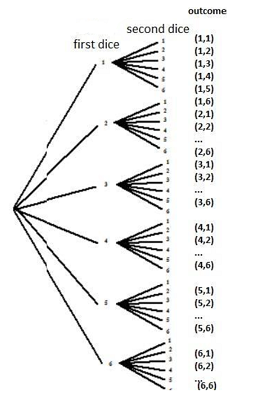The sample space containing the 36 sample points when rolling two dices are,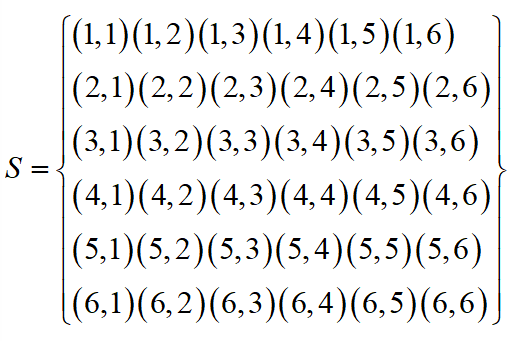Thus, the 36 sample points are stated.

Step 2

(b)

The probabilities assigned to each of the 36 sample points are,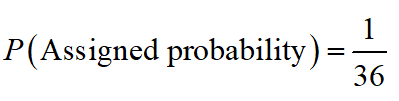Thus, each sample point has a probability 1/36.

Step 3

(c)

The probability of event A, 3 showing on each die is,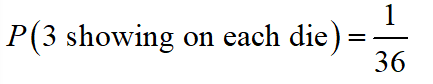Thus, the probability of 3 showing on each die is 1/36.

...

### Want to see the full answer?

See Solution

#### Want to see this answer and more?

Solutions are written by subject experts who are available 24/7. Questions are typically answered within 1 hour.*

See Solution
*Response times may vary by subject and question.
Tagged in